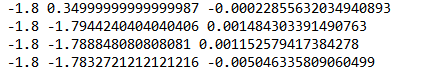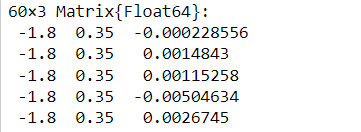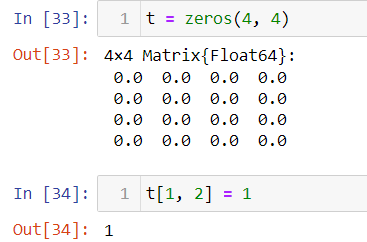# Convert data from txt file to matrix

Hello, I have txt file the following formatIt three column with numbers. How can i read data from file and convert him to matrix [N1, N2, 1] where N1 length first column, N2 length second column and 1 is the number that matches for example -1.8 and 0.34999999999999987 i.e -0.00022855632034940893 ( this example from image). Also how can I generalize this to the matrix [N1, N2, 4] for another text file where 6 columns.

Try

using DelimitedFiles

1 Like

Thank you,I tried using this package and get next result:this is a little not what I need.

I need a similar matrixIt is not very clear what your target is. From your first screenshot, it looks that you have a file with N rows, each row having three columns. You can read that as a N \times 3 matrix, which is what @pdeffebach suggested.

You are saying that you want an array of shape (N1, N2, 1), where N1 and N2 are the lengths of the first and second column, respectively, which is confusing since all columns have the same number of rows in your file.

Also, (N1, N2, 1) means that the last dimension is singleton, i.e., it can hold just a single value, and so (if a three-dimensional array is not required) it is essentially the same as an array of shape (N1, N2). Are you sure you want that?

Yes, i fixed the screenshot

And what adds to the confusion (I had exactly the same) is that in the screen-shot first and second columns are equal. Is this file supposed to contain x y z where x and y are some sort of coordinates?

Yes, x and y are coordinates

So, why are they repeated, and so many times?

I attached a screenshot from the wrong text file, I fixed it everywhere. Sorry.

I do not understand what the problem is. It doesn’t look like you did what I suggested in your notebook.

First and second column are coordinates and need get matrix [N, N] where value take from third column

Is your question how to read in the data or how to transform the data you read in to the shape you want? They are different steps.

How to transform the data to need shape

I think I know what is asked but we need to know one more thing. Is the data already gridded? i.e. are points regularly spaced or still needs to be gridded (interpolated)?

Find unique values of first column and sort. The length of that is N1. Create a Dict mapping those unique values to their index in the sorted vector. Do the same for the second column. The number of unique values in N2. Create a matrix N1xN2. Loop through rows looking up the matrix row index (r) from the first Dict using the first column value and matrix column index (c) from the second Dict using the second column value. Set the matrix element at r,c to the third column value.

Sorting is optional, but it is probably what you want.

Data is gridded. I can explain how this data is obtained, maybe it will help.

@joa-quim
I have started, ended value x and length range X = N. I create range for X from x_start to x_end with length = N. After this i get step from this range by range.step.

On the first step i calculate value y by function Y(x). Then i move to the right to x_end with value y i.e i fixed y and move to x_end with range.step.

On the second step i take next value x and calculate y(x), after this i move to the left to x_start with step = -range.step, y(x) is fixed. After this i move to right i.e to x_end with step = range.step, y(x) is fixed.

On the last step i.e x = x_end calculating y(x) and moving to the left i.e x_start

that is, I take a point x from the range, calculate the value of y(x) from it and move with a fixed y(x) to the left and right borders and so on to the very last value of x from the range.
and at each such point, some calculations are still performed, which gives the third column

OK, so you may be lucky and the following GMT.jl command will figure everything out. First, rename the file to have a *.xyz extension. Then do

using GMT

G = grdconvert("/path/to/yourfile.xyz")


And you get a full grid type (origin, increments, coordinates vector, etc…).

If that fails, the solution I know is to use the xyz2grd module but that requires consulting the manual on the original GMT version because I’ve not ported that manual to Julia usage yet. Though some help is provided with ? xyz2grd.

1 Like

Thank you!